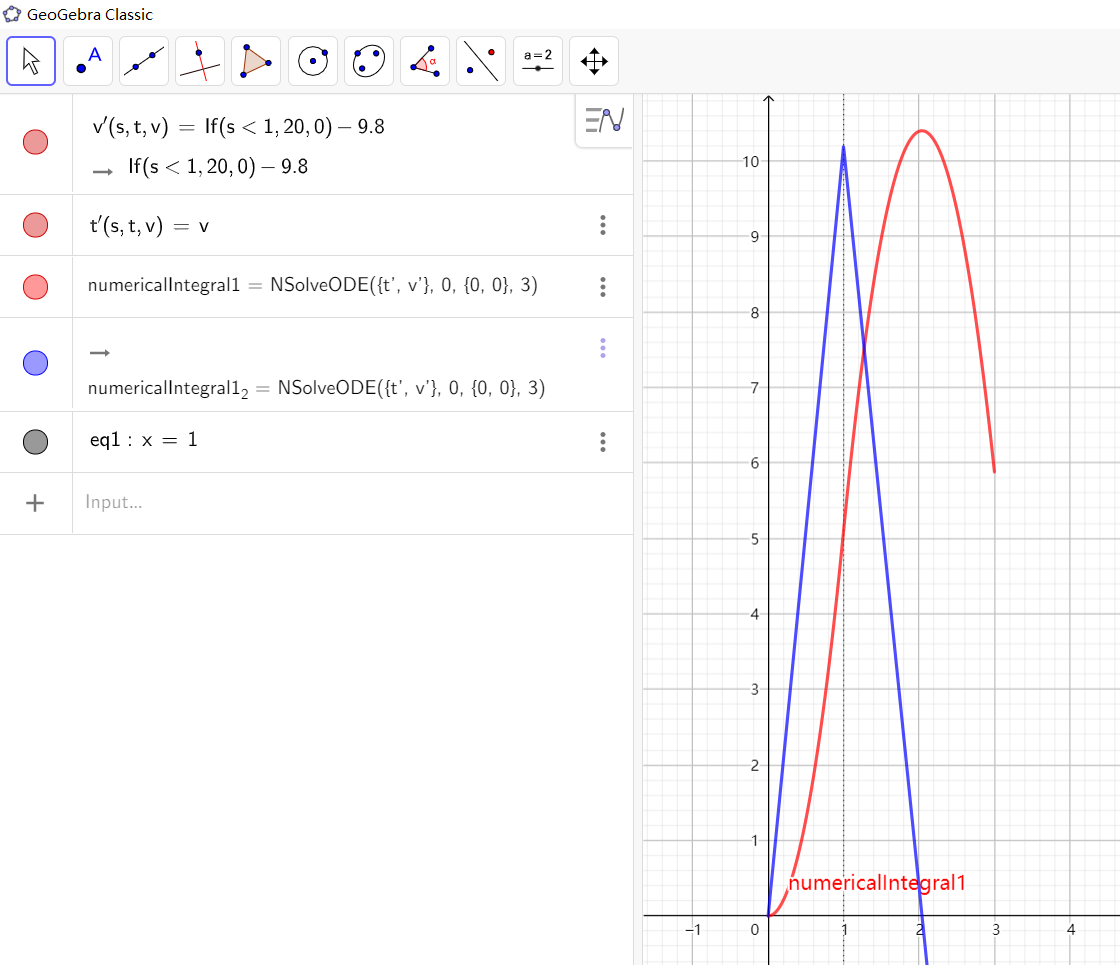# Using If while using NSolveODE

chrtsta shared this question 5 years ago

Hi,

We are working with model rockets, and wish to simulate a model rocket launch straight upwards. The reason to use a numerical tool for this is that we wish to include the air drag, which is not easy to do using only analytical tools.

What we wish to do is to include three forces: the thrust from the motor which is only non-zero for, lets say, the first second, the gravity and the air drag. We also use that the sum of forces is acceleration times the mass. Also, of course, the acceleration is the derivative of the velocity, and the velocity is the derivative of the position. Hence, we have a system of two differential equations, which we seemingly can solve using the NSolveODE function.

The problem is how we can model the thrust force. Let's not focus on the air drag right now, as that's not the problem. I have tried to simplify this a bit to make it easier to see the problem and to recreate it.

Running these three commands:

v'(x, v, r) = If(x < 1,50,0)-9.81

r'(x, v, r) = v

vr = NSolveODE({v', r'}, 0, {0, 0}, 3)

We get the attached plot. From the NSolveODE we of course get two functions (since that is the input). What makes vr1 look like that (which is the velocity, I think)? I would expect that from 0<x<1 it should have been increasing linearly, and for x>1 it should be decreasing linearly.

As you can see, vr1 increases linearly until about x=0.2, then it decreases linearly until about x=2, then increases linearly again to x=2.45 and decreases until it stops at x=3.

Also, playing around with the value of 50 in the If function makes it behave totally different. At low values (~5) the plots are exactly how I would expect.

Any help on this is appreciated!2Looks no problem.